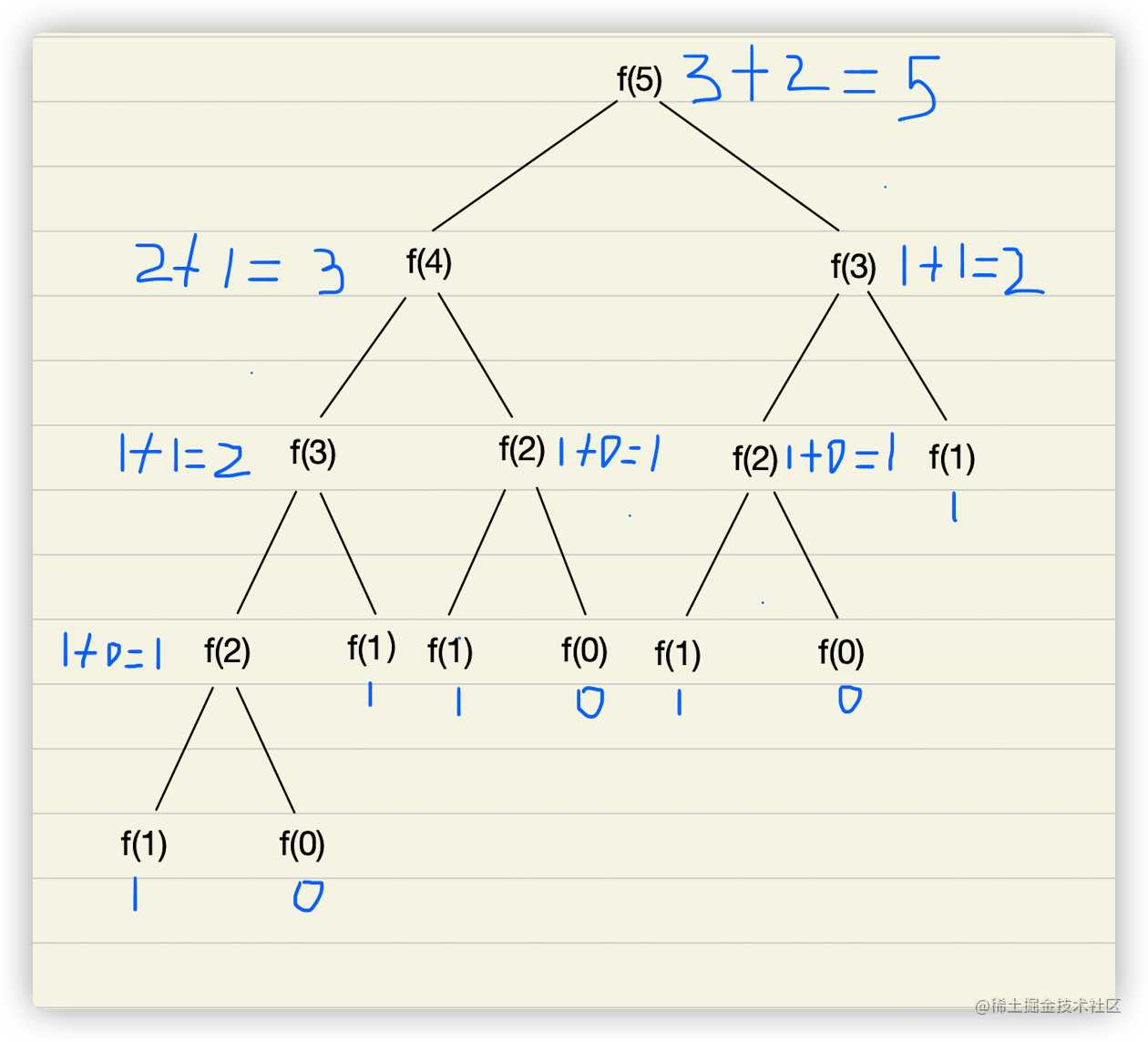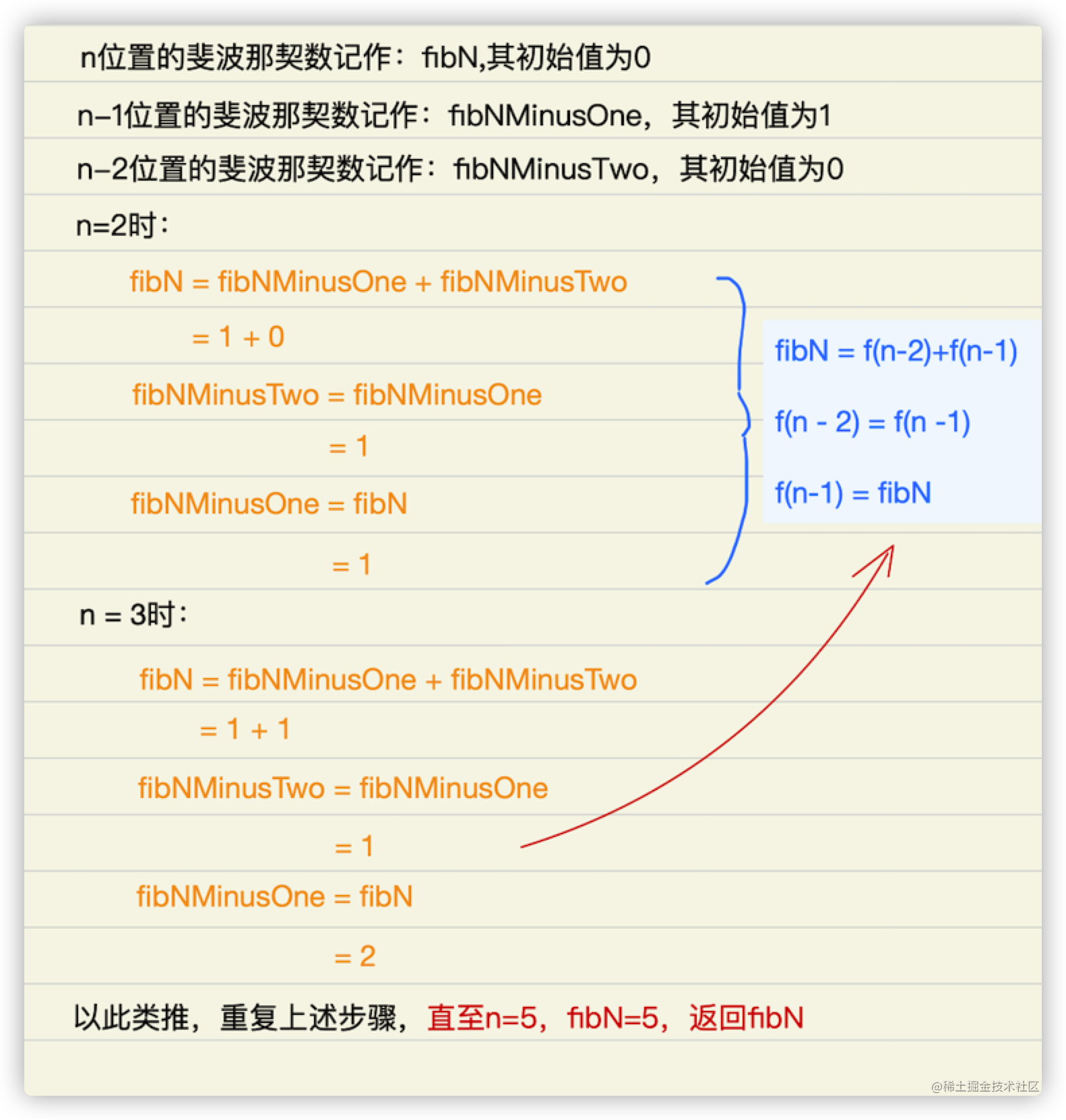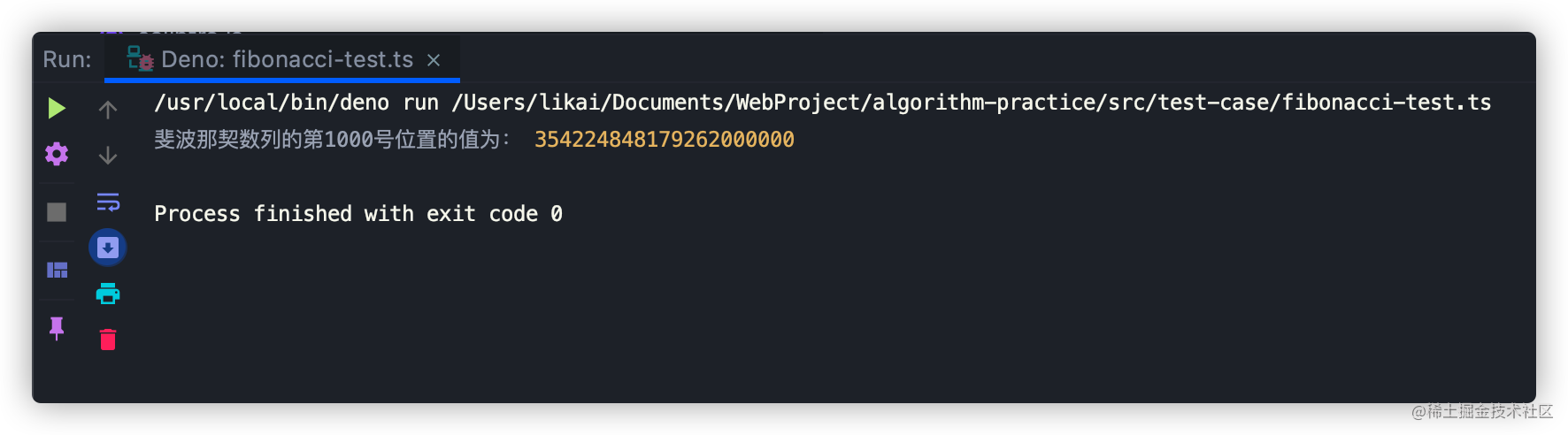• 累计撰写 165 篇文章
• 累计创建 25 个标签
• 累计收到 210 条评论

### 目 录CONTENT# 斐波那契数列的多种解法2021-06-23 / 0 评论 / 1 点赞 / 444 阅读 / 1,733 字

## 概念

• 4号位置的斐波那契数为 f(4-1) + f(4-2)
• 3号位置的斐波那契数为 f(3-1) + f(3-2)
• 2号位置的斐波那契数为 f(2-1) + f(2-2)
• 1号位置的斐波那契数为 1
• 0号位置的斐波那契数为 0

• 2号位置的斐波那契数就为：1 + 0 = 1
• 3号位置的斐波那契数就为：1 + 1 = 2
• 4号位置的斐波那契数就为：2 + 1 = 3
• 5号位置的斐波那契数就为：3 + 2 = 5

## 解决方案

### 递归解决### 自下而上## 实现代码

export default class Fibonacci {
constructor(index: number) {
this.index = index;
}

/**
* 自下往上实现
* 实现思路：
*   1. 根据f(0)和f(1)算出f(2)
*   2. 再根据f(1)和F(2)算出f(3)
*   3. 以此类推算出第n项
* 时间复杂度分析：它从第0项遍历到最后一项，因此时间复杂度为O(n)
*/
public bottomUp(): number {
const result: Array<number> = [0, 1];
if (this.index < 2) {
return result[this.index];
}

// f(n - 1)
let fibNMinusOne = 1;
// f(n - 2)
let fibNMinusTwo = 0;
let fibN = 0;
for (let i = 2; i <= this.index; ++i) {
// f(n) = f(n - 1) + f(n - 2)
fibN = fibNMinusOne + fibNMinusTwo;

// f(n - 2) = f(n - 1)
fibNMinusTwo = fibNMinusOne;
// f(n - 1) = f(n)
fibNMinusOne = fibN;
}
return fibN;
}
}


import Fibonacci from "../Fibonacci.ts";

const fibonacciTest = new Fibonacci(100);
console.log("斐波那契数列的第1000号位置的值为：", fibonacciTest.bottomUp());## 写在最后

• 文中如有错误，欢迎在评论区指正，如果这篇文章帮到了你，欢迎点赞和关注😊
• 本文首发于掘金，未经许可禁止转载💌
1